# The Beauty of Complex Analysis

Complex Analysis is the branch of mathematics that studies functions of complex numbers. These numbers are those given by a + bi, where i is the imaginary unit, the square root of -1. We can think of complex numbers as points in a plane, where the x coordinate indicates the real component and the y coordinate indicates the imaginary component.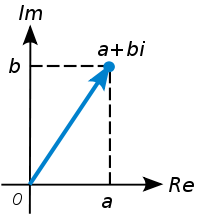From that, we can consider functions of complex variable as maps that take each point in the plane to another point in the plane.

There is one characteristic of Complex Analysis that makes it especially beautiful. Inside of it we can find objects that appear to be very complicated but happen to be relatively simple. Reciprocally, there are objects that appear to be very simple but are indeed extremely complex.

For example, in Complex Analysis we study holomorphic functions. These are essentially functions that are differentiable in the complex sense. Which means that the function is continuous and both its real and imaginary parts have partial derivatives that satisfy the (apparently simple) Cauchy-Riemann equations. At first sense this is a very general definition. Considering the huge variety of differentiable functions in the real line we would imagine that there is also an extreme variety of holomorphic functions in the plane. However in complex analysis we have for example the following theorems, which impose very strong conditions on holomorphic functions:

• If a function is holomorphic in the entire plane and its bounded in module, then its constant. This is Liouville's_theorem and it actually proves the Fundamental Theorem of Algebra
• If we know the value of a holomorphic function in a circle, then we can know the value in every single point inside of the disk, by the Cauchy Integral Formula
• If two holomorphic functions are equal in an infinite number of points (with one accumulation point) then they are equal in a complete disk, this is because they are analytic

The last point is particularly shocking when one compares it with real functions. For example, the function {0 if x < 0 and exp(-1/x) if x >= 0} is identically equal to the constant 0 on the left side of the graph, and both are infinitely differentiable. However, they are obviously different functions. As a matter of fact, this function is a counterexample to show that the 3 properties above don't apply to real functions.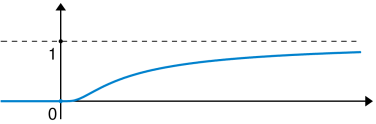Holomorphic functions are very structured when compared with real differentiable functions. In that sense they are more simple than what intuition would suggest.

On the other hand, we have objects like the Mandelbrot set, which can be expressed in a very simple and concise way, but which is indeed very complex. From Wikipedia: A complex number c is in the Mandelbrot set if, when starting with z0 = 0 and applying the iteration: z_n+1 = (z_n)^2 + c the absolute value of z_n never exceeds a certain boundary, however large n gets. In spite of this simplicity, the graphical representation of a part of this set looks like this: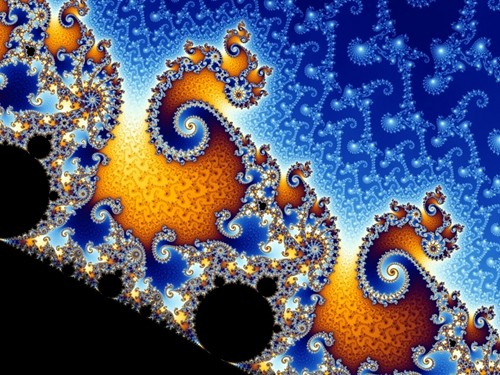Another example, even more interesting: Consider the Riemann Zeta Function: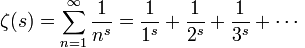where s is a complex parameter. This function can be defined in a seemingly very simple way. However it satisfies many remarkable properties. For instance, if we restrict ourselves to the stripe of the complex plane where the real component x is < x < 1 and take a section of the stripe and any holomorphic function which doesnt take the the value 0 we have that, informally, this function can be approximated by the zeta function as precisely as we want in another section of the strip right above or below. This property is called the Universality of the Riemann Zeta Function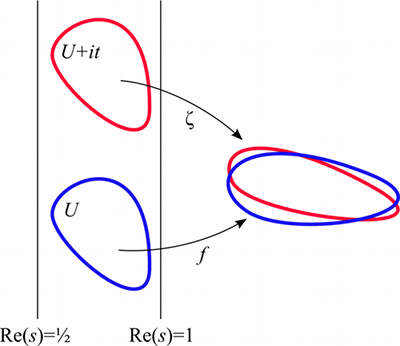Think about that, it doesnt matter how complex an holomorphic function is inside of that area. As long as it doesnt become 0, there is another area in which the zeta function approximates it as precisely as we want. In that sense the structure of the zeta function is so rich that it contains within itself the shape of every other non-vanishing holomorphic function.

Written on January 11, 2011